Two months

Mars has two months, Phobos and Deimos. Phobos orbits around Mars in 7 hours 39 minutes and Deimos in 30 h 14 min. How long will repeat the relative position of the three celestial bodies?

Result

t = 13877:06Solution:Leave us a comment of this math problem and its solution (i.e. if it is still somewhat unclear...):Be the first to comment!To solve this verbal math problem are needed these knowledge from mathematics:

Do you want to calculate least common multiple two or more numbers?

Next similar math problems:

1. Trams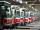Two trams started at the same time from the same place. One tram journey takes 30 minutes and the second 45 minutes to its final stop. How long will trams meet again?
2. Days2minutesWrite a formula that converts x days to y minutes. The formula is:
3. Theorem proveWe want to prove the sentence: If the natural number n is divisible by six, then n is divisible by three. From what assumption we started?
4. Nuts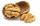How many we must have at least nuts if we can equally divide it to 10 children, 11 children or 19 children and any nut left?
5. Seamstress 2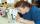Seamstress bought two kinds of textile in whole meters. One at 50 SKK and the second 70 SKK per meter. How many meter bought from this two textiles when paid totally 1540 SKK?
6. Numbers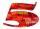Write smallest three-digit number, which in division 5 and 7 gives the rest 2.
7. Plum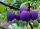On the platter are plum. How many were there if its have to be able to share equally among 8,10 and 12 children?
8. Factory and divisionsThe factory consists of three auxiliary divisions total 2,406 employees. The second division has 76 employees less than 1st division and 3rd division has 212 employees more than the 2nd. How many employees has each division?
9. Linear systemSolve a set of two equations of two unknowns: 1.5x+1.2y=0.6 0.8x-0.2y=2
10. FamilyFamily has 4 children. Ondra is 3 years older than Matthew and Karlos 5 years older than the youngest Jane. We know that they are together 30 years and 3 years ago they were together 19 years. Determine how old the children are.
11. PowersExpress the expression ? as the n-th power of the base 10.
12. Two equationsSolve equations (use adding and subtracting of linear equations): -4x+11y=5 6x-11y=-5
13. Father 7Father is 6 times older than his son. After 4 years, the father will only be 4 times older. What are their present ages?
14. 6 termsFind the first six terms of the sequence. a1 = 7, an = an-1 + 6
15. Jane plantsJane plants flowers in the garden. If she planted 12 every hour instead of 9 flowers, she would finish with the job an hour earlier. How many flowers does she plant?
16. Volleyball8 girls wants to play volleyball against boys. On the field at one time can be six players per team. How many initial teams of this girls may trainer to choose?
17. Ages 2A man's age is 4 times his son's age. After 5 years he will be just twice his son's age, find their ages.